# Catch up on the latest AI articles# Self-Supervised Material Texture Representation Learning For Remote Sensing

3 main points
✔️ We proposed a novel material texture-based self-supervised learning method to obtain features with the high inductive bias required for downstream tasks on remote sensing data.
✔️ The models pre-trained with our method recorded SOTA in supervised and unsupervised change detection, segmentation, and land cover classification experiments.
✔️ Provided a multi-time spatially adjusted, atmospherically processed remote sensing dataset in an unchanging domain used for self-supervised learning.

written by Peri AkivaMatthew PurriMatthew Leotta
(Submitted on 3 Dec 2021)

Subjects: Computer Vision and Pattern Recognition (cs.CV); Artificial Intelligence (cs.AI); Image and Video Processing (eess.IV)code：The images used in this article are from the paper, the introductory slides, or were created based on them.

## first of all

Self-supervised learning aims to learn the representation features of an image without annotation data. In addition, by initializing the network weights to the weights learned by self-supervised learning in a downstream task, faster convergence and higher performance may be obtained. However, self-supervised learning requires a high inductive bias. In this paper, we proposed material-texture-based self-supervised learning called MATTER (MATerial and TExture Representation Learning).In MATTER, to obtain the luminance and viewing angle invariants, multiple spatially adjusted remote sensing data in time to obtain luminance and viewing angle invariants.

## technique

A schematic diagram of MATTER is shown below. Given an anchor image $x_a\in {\cal R}^{B\times H \times W}$ in an unchanged region, we obtain a positive image $x_p\in {\cal R}^{B\times H \times W}$ in the same region and a negative image $x_n\in {\cal R}^{B\times H \times W}$. Where $B, H, W$ are the number of bands, height, and width of the input image. Tile all the images into P patches of size $H\times W$ and denote them as $c_a, c_p, c_n$ respectively. To learn material-texture-centric features, we propose a Texture Refinement Network (TeRN) and a patch-wise Surface Residual Encoder.TeRN aims to increase the activation of low-level features required for the texture representativeness Surface Residual Encoder is a patch-wise adaptation of prior work Deep-TEN to learn surface-based residual representation quantities. The network is trained by minimizing the feature distance of positive patch pairs $c_a, c_p$ and maximizing the feature distance of negative patch pairs $c_a, c_n$. Here the features are the learned residual representation quantities. We used the noise-contrast loss as the objective function.

$${\cal L}_{NCE}=-{\mathbb E}_C\left[{\log}\frac{\exp (f(c_a)\cdot (f(c_p))}{\sum_{c_j \in C}\exp (f(c_a)\cdot f(c_j))}\right]$$

where $f(c_j)$ is the feature of patch $c_j$ and $C$ is the set of positive and negative patches.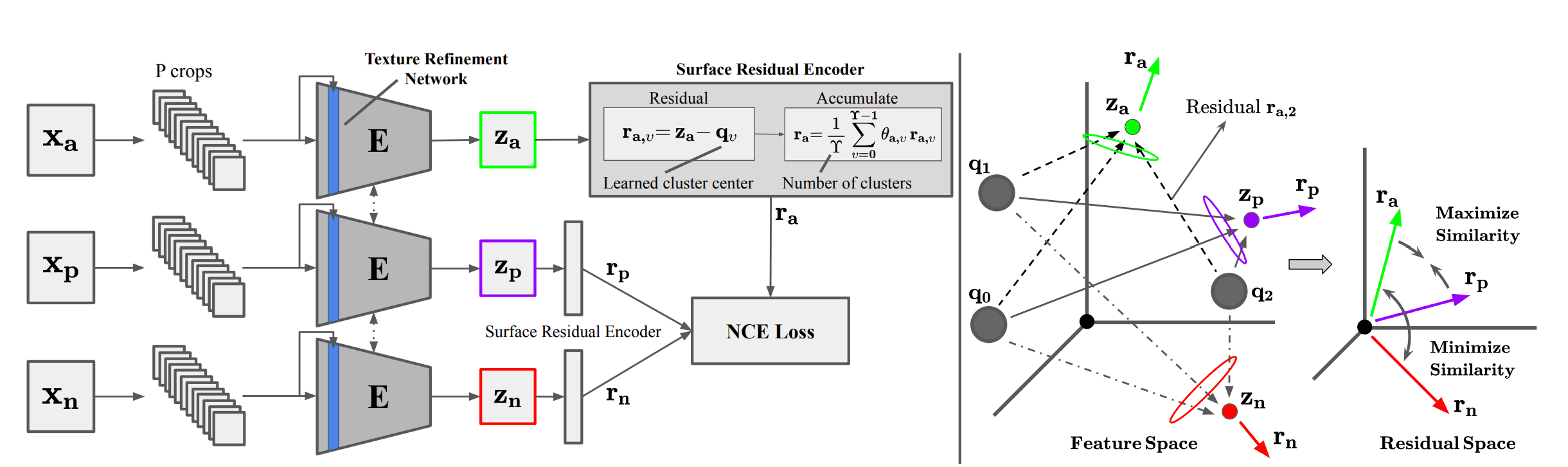### Texture Refinement Network

TeRN refines texture features, which are often low-level in satellite images. It employs the recently proposed pixel adaptive convolution layer, and given a kernel $k^{i,j}$ centered at position $(i,j)$, calculates the cosine similarity between pixel $x_{i.j}$ and the neighborhood point ${\cal N}(i,j)$, and divides by the square of the standard deviation $\sigma_{{\ cal N}(i,j)}$ and divide by the square of $\sigma_{{\cal N}(i,j)}$.

$$k^{i,j}=-\frac{1}{\sigma^2_{{\cal N}(i,j)}}\frac{x_{i,j}\cdot x_{p,q}}{||x_{i,j}||_2\cdot ||x_{p,q}||_2}, \forall p,q \in {\cal N}(i,j)$$

The above equation represents the similarity to the center pixel and the gradient strength in the kernel. Since the texture represents the spatial distribution of the structure, it is directly related to the gradient strength. A single kernel layer is $K$ and a refinement network of $L$ layers is constructed.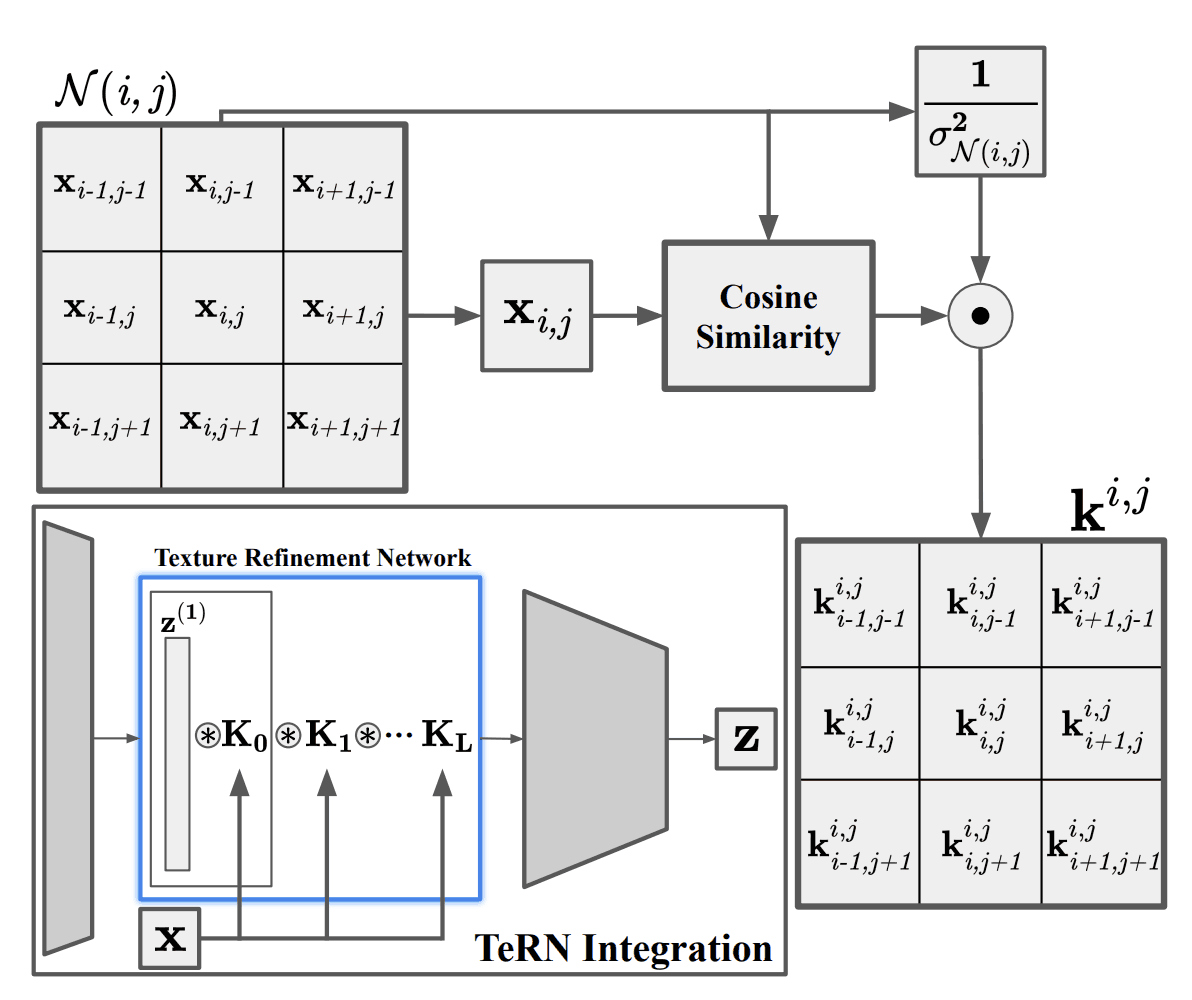## Surface Residual Agreement Learning

This method performs patchwise clustering. It learns residuals of small patches and enforces consistency with the corresponding multi-time patch residuals. Given a feature vector $z_i^{1\times D}$ and $\Upsilon$ learned cluster centers $Q=\{q_0,q_1,\cdots,q_{\Upsilon-1}\}$ for some crop $c_i$, the residuals between $z_i$ and cluster center $q_v$ are $r_{i,v}^{1\times D}=z_i-q_v$. By repeating this for all clusters and taking the weighted average, the final residual vector is obtained as follows.

$$r_i = \frac{1}{\Upsilon}\sum_{v=0}^{\Upsilon-1}\theta_{i,v}r_{i,v}$$

where $\theta_v$ is the learned cluster weights. By using this, we can consider the affinity between clusters.

## experiment

### prior learning

For self-supervised learning, we used ortho-rectified, atmospherically processed Sentinel-2 data from a non-urbanized region. A total of $1217 km^2$ of $27$ area was finally collected, yielding $14857$ $1096^times 1096$ tiles.

### change detection

We used the Onera Satellite Change Detection (OSCD) dataset. We evaluated two types of models: a self-supervised learning model with only a pre-training model and a supervised model that was fine-tuned with the supervised data afterward. The change point was the case where the Euclidean distance between the residual features of the before and after images exceeded a threshold value. The results are shown in the table below. The F1 score recorded SOTA for both self-supervised and supervised models.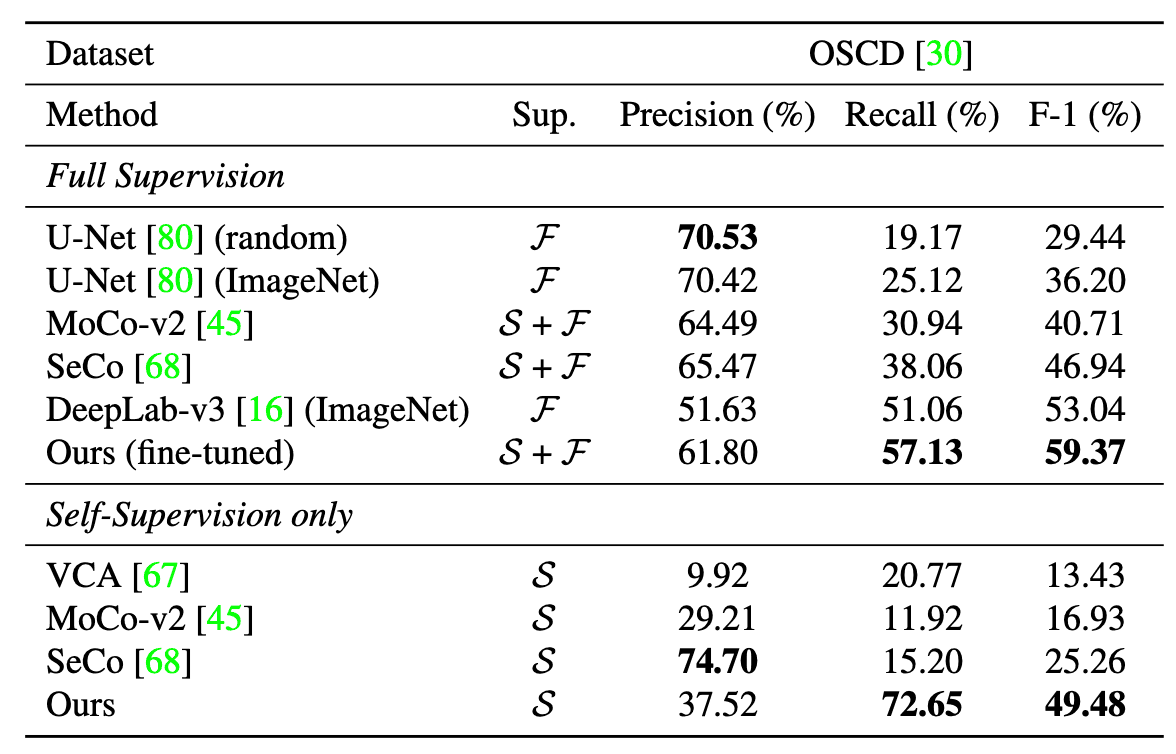### land subsidence classification

As a dataset, we used the BigEarthNet dataset with 19 classes of land cover labels. The results are shown in the table below. The method recorded an average accuracy SOTA.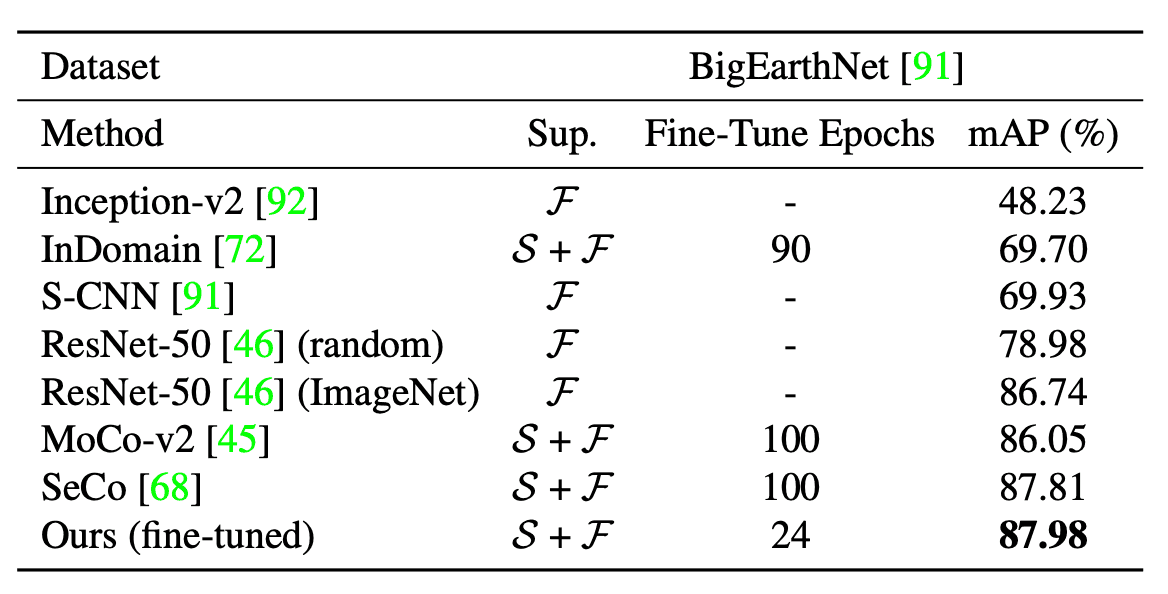### segmentation

As a dataset, we used SpaceNet for building segmentation. The results are shown in the table below. The method recorded a SOTA of average IoU.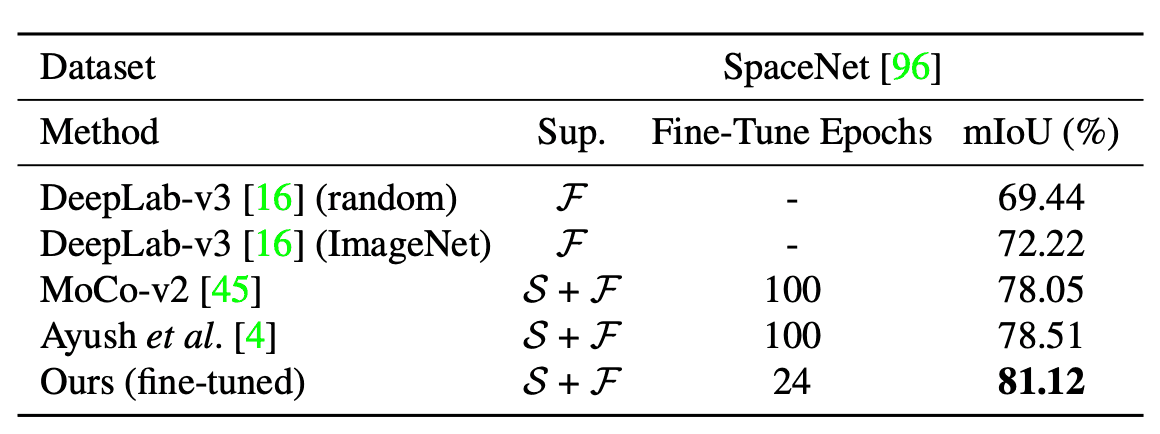## result

By implementing our method, we achieved faster convergence and higher accuracy for downstream tasks and found that texture and material are important features. We also compared the visual word map (pixel-wise clustering) to qualitatively evaluate whether the material and texture are well represented. The results are shown in the figure below: Textons evaluate pixel values and are therefore more sensitive to small changes in texture, leading to multiple clusters of the same class; Patch-wise Backbone loses information from low-level features, leading to multiple classes being clustered into a single cluster. On the other hand, our method classifies very close to the input image.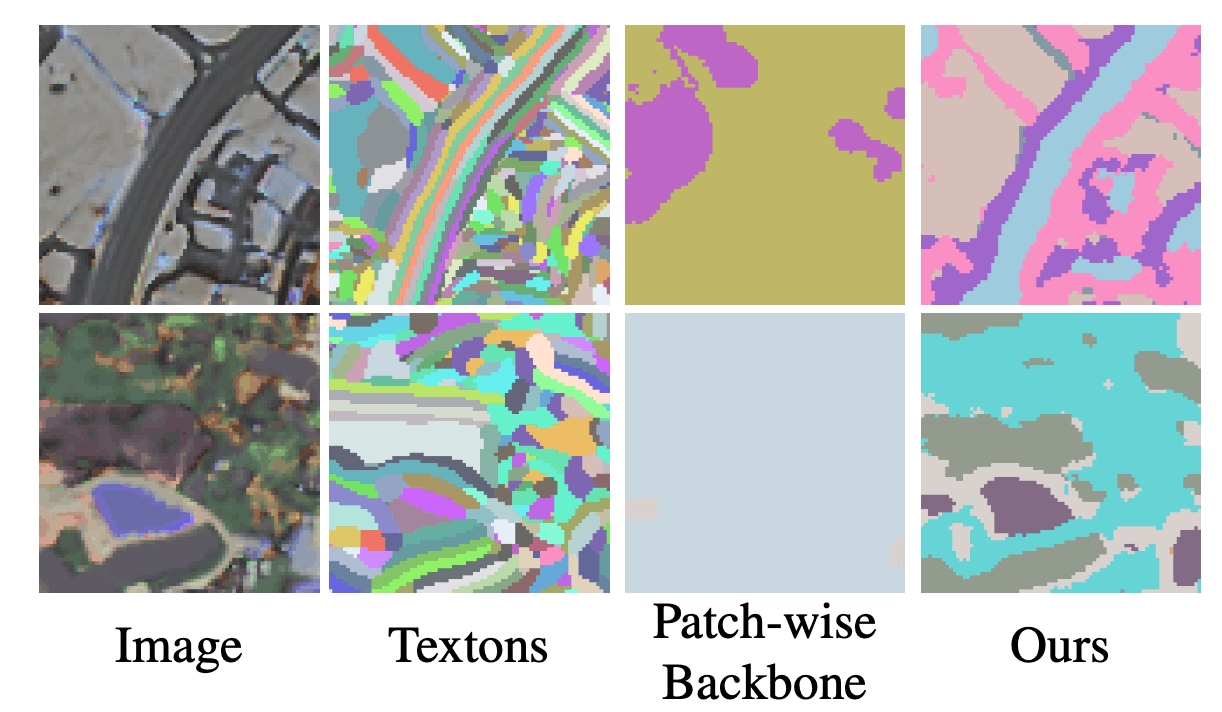## summary

In this paper, we proposed a self-supervised learning method called MATTER, which learns texture and material features that are strongly correlated with surface changes and could be applied to pre-training for remote sensing tasks.

If you have any suggestions for improvement of the content of the article,